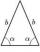# Cosine + real numbers - problems

1. Trigonometric functionsIn right triangle is: ? Determine the value of s and c: ? ?
2. Regular 5-gonCalculate area of the regular pentagon with side 7 cm.
3. ForcesIn point O acts three orthogonal forces: F1 = 20 N, F2 = 7 N and F3 = 19 N. Determine the resultant of F and the angles between F and forces F1, F2 and F3.
4. IS triangleCalculate interior angles of the isosceles triangle with base 38 cm and legs 26 cm long.
5. CuboidDetermine the dimensions of cuboid a, b, c; if diagonal d=9 dm has angle with edge a α=55° and has angle with edge b β=58°
6. Triangle TBCTBC is isosceles triangle with base TB with base angle 63° and legs length |TC| = |BC| = 25. How long is the base TB?
7. CotangentIf the angle α is acute, and cotg α = 1/3. Determine the value of sin α, cos α, tg α.

We apologize, but in this category are not a lot of examples.
Do you have an interesting mathematical problem that you can't solve it? Enter it, and we can try to solve it.

To this e-mail address, we will reply solution; solved examples are also published here. Please enter e-mail correctly and check whether you don't have a full mailbox.